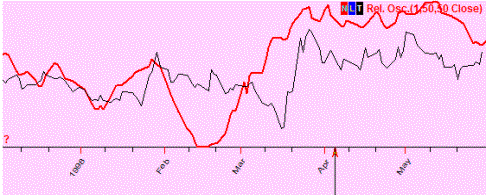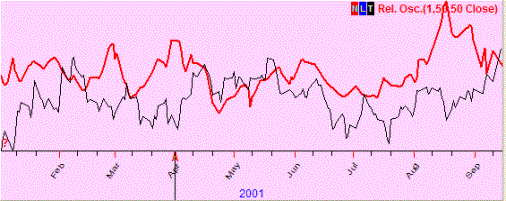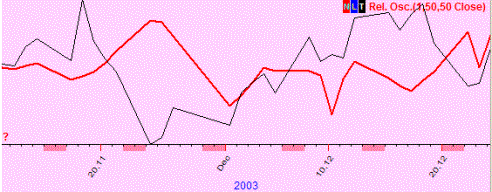What is the Correlation Coefficient?

This is the definition from Financial Forecast Center (http://www.neatideas.com/cc.htm).

What is the Correlation Coefficient?

The correlation coefficient concept from statistics   is a measure of how well trends in the predicted values follow trends in the actual values in the past.  It is a measure of how well the predicted values from a forecast model "fit" with the real-life data.

The correlation coefficient is a number between 0 and 1.  If there is no relationship between the predicted values and the actual values the correlation coefficient is 0 or very low (the predicted values are no better than random numbers).  As the strength of the relationship between the predicted values and actual values increases so does the correlation coefficient.  A perfect fit gives a coefficient of 1.0.  Thus the higher the correlation coefficient the better.

The correlation coefficient varies in the range of  -1 (-100%)..+1(+100%). Timing Solution software measures the correlation coefficient in %%. It means that instead of r=0.123 we use r=12.3%

Practice shows that for financial analysis it is better to use percentage. For example, suppose we have found the projection line that provides correlation 5%. Following the usual notation, it should be marked as r=0.05.

It can be easily misread as r=0.005, especially when you analyze many correlations (like in Walk Forward Analysis reports).

If you use percentage notation, it is r=5% versus r=0.5%, you never mix them up. It is a convenient way as 5% level is very important (see below the explanation).

What correlation is good enough? The more the better. Usually, the models that we analyze provide 10%-20% correlation. Sometimes it is more than that, but these results are not stable. To be sure that the result is not accidental, it is necessary to have a sufficient amount of price bars for calculating the correlation. For practical usage, you can use this table:

 Correlation Meaning 100% means ideal coincidence between some data and the projection line 5% weak correlation, this projection line is not usable though it reflects some stock market movements 10% we can take it as a not bad correlation 15% good correlation 0% no correlation. Two sets of data are not related -100% this is anti-correlation, which means that the predicted values "mirror" the actual values (or one data set is the "mirror" for another one).

These are examples:

Positive correlation (50%); these two curved lines show the same price movement (most of the time). In other words, a predicted price and the actual one show the same price movement (up or down):No correlation (7%); these two curved lines show totally different movements (if one goes up, the other may go up or down and there is no regularity seen there):Negative correlation (-4%); we observe the "mirror" effect (when one curved line goes up, the other one goes down in most cases, and vice versa):